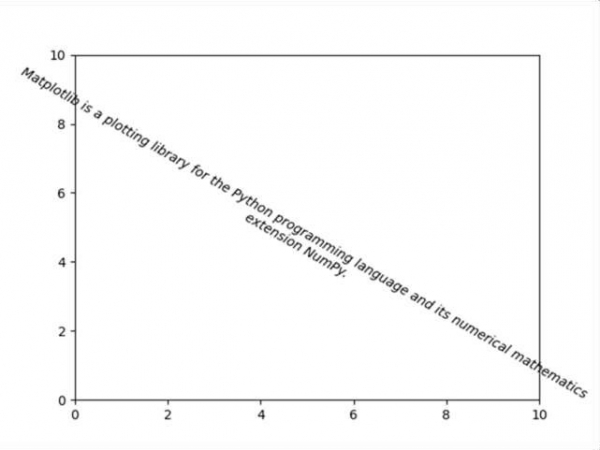# Text box with line wrapping in Matplotlib

Matplotlib can wrap text automatically, but if it's too long, the text will be displayed slightly outside of the boundaries of the axis anyways.

## Steps

• Create a new figure, or activate an existing figure, using figure().

• Set the axis properties using plt.axis() method.

• Make a variable input_text to store the string.

• Add text to figure, using plt.text() method where style='oblique', ha='center', va='top', ...etc.

• To show the figure use plt.show() method.

## Example

import matplotlib.pyplot as plt

fig = plt.figure()
plt.axis([0, 10, 0, 10])
input_text = 'Matplotlib is a plotting library for the Python programming
language and its numerical mathematics extension NumPy.'
plt.text(5, 5, input_text, fontsize=10, style='oblique', ha='center', va='top', wrap=True, rotation=-30)

plt.show()

## Output